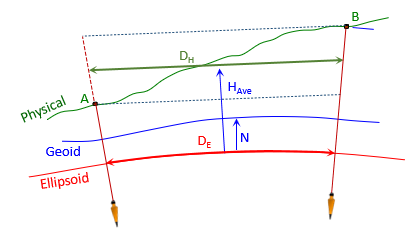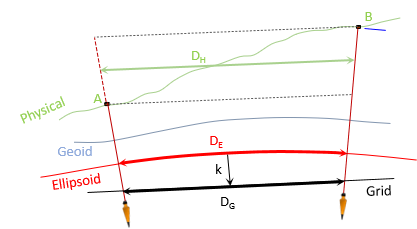### 1. Preliminary Calculations

#### a. Survey Data

This chapter covers measurements reduction for an SPC conic zone. Distances and coordinates will be in survey feet.

The survey data and SPC grid information for point Jerry are summarized in Figure K-1 and Table K-1.Figure K-1 Survey Data

 Table K-1 Orthometric Heights Point H, ft Jerry 1177 A1 1805 B7 1340

#### b. Approximate Coordinates

Compute approximate SPC coordinates of A1 and B7.
Point A1Point B7### 2. Ground Distance to Grid

#### a. Ground to Ellipsoid

Going from ground to the ellipsoid is independent of the grid system.Figure K-2 Ground to Geodetic

Use Equation H-2 to reduce ground to the ellipsoidEquation H-2
Te equation uses a line's average elevation and the average geoid height to determine the geodetic length on the ellipsoid.
RE is 20.906 x 106 ft.

Jerry's geoid height is -33.902 meters computed using the GEOID18 model. It is negative because the geoid is below the ellipsoid in Wisconsin.
Since we're working in feet, the geoid height must be converted from meters:Geoid height generally does not vary significantly over an area of this size. Since Jerry is centrally located, -111.2 ft can be used as a project average.

##### (1) Geodetic distance Jerry-A1##### (2) Geodetic distance Jerry-B7#### b. Ellipsoid to GridFigure K-3 Geodetic to Grid

To go from ellipsoid to grid, the geodetic distance multiplied by the grid scale factor, k, Equation H-3.Equation H-3

The grid scale in Equation H-3 can be:

Jerry's scale used for the entire project
Average of the endpoint scales for each line
A weighted average scale, Equation H-4, for each line based on scales at the mid- and endpointsEquation H-4

We'll compute the grid distance each way and compare their results.

##### (1) Using Jerry's scale: k=0.99996 957.
 Line Grid Distance Jerry-A1:Jerry-B7:##### (2) Average scale for each line

Using software, scale at points A1 and B7 can be determined using their approximate coordinates. NGS's NCAT can be used for this as well as the NAD 83 Coordinate Conversion workbook.

 Point Scale A1 0.99996 8421 B7 0.99996 8894

Multiplying each line's geodetic distance by its average scale:

 Line Average k Grid Dist Jerry-A1 0.99996 8996 4281.768 Jerry-B7 0.99996 9232 5145.760
##### (3) Weighted average scale for each line

Use the same software to determine the scale at each line's midpoint; midpoint coordinates are the average of the endpoint coordinates.

 Line Mid-point k Jerry-A1 0.99996 8991 Jerry-B7 0.99996 9230

Multiplying each line's geodetic length by its weighted average scale:

 Line Weighted k Grid Dist Jerry-A1 0.99996 8992 4281.768 Jerry-B7 0.99996 9231 5145.760
##### (4) Combined Factor

Grid distance can also determined from multiplying ground distance by Jerry's Combined Factor: 0.99991 863, Equation H-6.Equation H-6

This simplified method does not require computing geodetic distances - the entire project is scaled by a single CF.

 Line Grid Dist Jerry-A1Jerry-B7##### (5) Comparing results

Theoretically, the weighted average scale gives the best grid distance. In Table K-2 it is used as the base to which the others are compared.

 Table K-2 Grid Reduction Comparisons Jerry-A1 Jerry-B7 DE x k Grid Dist, ft Diff, ft Grid Grid, ft Diff, ft Wtd Ave k 4281.768 - - 5145.760 - - Average k 4281.768 0.000 5145.760 0.000 Jerry's k 4281.771 +0.003 5145.761 +0.001 Combined Factor DHxCF 4281.762 -0.006 5145.781 +0.021

The largest differences are for the CF grid distances. Considering there is a 255 foot elevation variation across the project, that's not surprising. The other differences are 0.003 ft or less. An acceptable level of accuracy might be achieved with Jerry's scale factor for all lines - it simplifies computations somewhat although using line averages increases the accuracy without too much more effort.

Depending on accuracy requirement, a worse-case scenario should be examined. Consider a line furthest from the control in direction of scale variation and/or at largest elevation difference. Any method acceptable for that line will be acceptable for the entire project.

### 3. Grid Direction

To convert the geodetic azimuth of line Jerry-A1 to grid, we need to know:

• where the two meridians are with respect to each other, and
• if the arc-to-chord correction should be applied

#### a. Meridians

Convergence is the angle from Geodetic N to Grid N. Jerry's SPC convergence is +0°11'03.9". Because it is positive, Geodetic N is west of Grid N, Figure K-4.Figure K-4 Geodetic and Grid North

Another way to determine the meridians' relationship is by comparing longitudes of Jerry and the CM. This requires knowledge of the zone parameters. The CMs of all three Wisconsin SPC zones are at 90°00'00"W longitude. Jerry's longitude is 89°43'53.76413"W which places it east of the CM. Because Jerry is east of the CM, Geodetic N west of Grid N, Figure K-5.Figure K-5 Longitudes

#### b. Arc-to-Chord

The arc-to-chord correction for line Jerry-A1 can be computed using either Equation H-9 or H-10. We'll use both to see if there's a difference.Equation H-9Equation H-10

Both equations require No which is the north coordinate of the CP at the CM. Even though a constant, it is not given as a zone parameter and must be computed using the projection equations. NCAT does not provide this information but the NAD 83 Coordinate Conversion workbook does.

Wis South SPC Zone No = 510,708.62 ft = 155,664.30 m
##### (1) Equation H-9

Because Equation H-9 is set up for metric coordinates,we'll need to convert point A1.Equation H-9 in terms of point identifiers:Coordinate differences:Substituting into the correction equation:#### (2) Equation H-10

Equation H-10 in terms of point identifiers is:This coordinates may be metric or Imperial. We'll stick to feet.

This equation requires ro which, like No, is constant for for a zone but not given as a defining parameter. NCAT does not provide this information but the NAD 83 Coordinate Conversion workbook does.

For Wis SPC South zone, ro = 20,920,156.06 ft.

Coordinate differences:Substituting into the correction equation:##### (3) Application

Both equations yield the same result (surprise, surprise).

Is the arc-to-chord correction significant? That's up to the surveyor and the type of project.  Even though it's less than a second, we'll apply it as it may affect rounding.

To compute the Grid Azimuth from Geodetic, convergence, and arc-to-chord correction, use Equation H-7.Equation H-7

A sketch can also be used to determine how to apply the correction. Because the North coordinates of Jerry and A1 are less than No, the line is south of the CP which makes it concave northerly toward the CP, Figure K-6.Figure K-6 Line Jerry-A1 Concavity

The arc-to-chord correction computed with Equations H-9 and H-10 is negative making it an angle to to the left which Figure K-6 supports. Figure K-7 shows all the elements to convert the Geodetic Azimuth to Grid.Figure K-7 Grid Azimuth

Using Jerry's convergence and a negative arc-to-chord correction, the Grid Az of Jerry-A1 is:### 4. Ground to Grid Angle

To convert angle B7-Jerry-A1 to grid, we need the arc-to-chord correction for lines Jerry-A1 and Jerry-B7. We have the former, now must compute the latter. Line Jerry-B7 is longer and oriented more east-west than Jerry-A1 so the magnitude of its arc-to-chord correction should to be larger.

We've shown that both arc-to-chord equations give the same results so we'll use Equation H-10.The correction for Jerry-B7 is larger than Jerry-A1's as expected.

At Jerry the correction to A1 is negative (angle left) and to B7 positive (angle right). The angle and corrections are shown in Figure K-7.Figure K-7 Angle Corrections

The grid angle B7'-Jerry-A1',  βG , is:The user must decide if combined bascksight and foresight arc-to-chord correction is significant.

### 5. Summary

This example looked at a few different SPC grid reduction methods. Depending on the accuracy needs of the survey, simple methods may be sufficient instead of more complicated (and time consuming) ones. The decision should be based on examination of worse-case scenarios.

If the two lines of the example survey represent the extreme situations, we determined that

• distance reduction - Jerry's grid scale could be used for the entire project but each line should use its own average elevation
• arc-to-chord correction - was not significant enough to affect directions or angles.